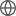Old Web
English
One of the most important discoveries to emerge from the ESA Gaia astrometric survey is the "phase spiral" pattern detected in the $z-V_z$ plane throughout the solar neighbourhood. In Galactic cylindrical coordinates ($R,\phi,z$),individual stars have velocities ($V_R,V_\phi,V_z$) and oscillation frequencies ($\Omega_R,\Omega_\phi,\Omega_z$)=($\kappa,\Omega,\nu$). The phase spiral is most evident when each point of the $z-V_z$ phase plane is represented by either $\langle V_R\rangle$ or $\langle V_\phi\rangle$, averaged over the volume, indicating that the disturbance has locked the vertical oscillations with the in-plane epicyclic motions. We revisit Binney & Schoenrich (2018) who use an analytic model of a disc-crossing satellite ($2\times 10^{10}$ M$_\odot$) to explain the phase spiral and its coupled behaviour in $\langle V_R\rangle$ and $\langle V_\phi\rangle$. With the aid of the AGAMA and RAMSES codes, we explore a high-resolution N-body model that is true to the analytic calculation. We find that the impulse triggers a superposition of two distinct bisymmetric (m=2) modes -- a density wave and a bending wave -- that wrap up at different rates. Stars in the faster density wave wrap up with time $T$ according to $\phi_D(R,T)=(\Omega_D(R)+\Omega_o)T$ where $\phi_D$ describes the spiral pattern and $\Omega_D=\Omega(R)-\kappa(R)/2$. While the pattern speed $\Omega_o$ is small, it is non-zero indicating that this is a dynamic density wave. The slower bending wave wraps up according to $\Omega_B\approx\Omega_D/2$ producing a corrugated wave, a phenomenon now well established in the Galaxy. The bunching effect of the density wave triggers the "phase spiral" as it rolls up and down on the bending wave ("rollercoaster" model). The phase spiral emerges slowly about 380 Myr after impact, and symbiotically in $\langle V_R\rangle$ and $\langle V_\phi\rangle$...